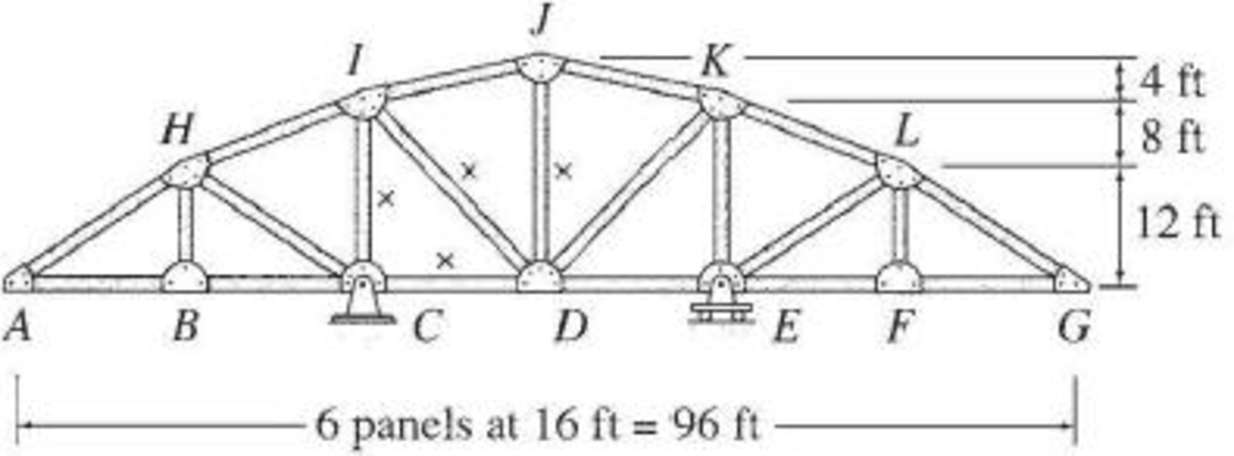# 8.45 through 8.52 Draw the influence lines for the forces in the members identified by an “×” of the trusses shown in Figs. P8.45–P8.52. Live loads are transmitted to the bottom chords of the trusses. FIG. P8.51

#### Solutions

Chapter
Section
Chapter 8, Problem 51P
Textbook Problem
26 views

## 8.45 through 8.52 Draw the influence lines for the forces in the members identified by an “×” of the trusses shown in Figs. P8.45–P8.52. Live loads are transmitted to the bottom chords of the trusses.FIG. P8.51To determine

Draw the influence lines for the force in member CD, CI, DI, and DJ.

### Explanation of Solution

Calculation:

Find the support reactions.

Apply 1 k moving load from A to G in the bottom chord member.

Draw the free body diagram of the truss as in Figure 1.

Refer Figure 1,

Find the reaction at C and E when 1 k load placed from A to G. (0x96ft).

Apply moment equilibrium at C.

ΣMC=01(32x)Ey(32)=032Ey=(32x)32Ey=x32Ey=x321

Apply force equilibrium equation along vertical.

Consider the upward force as positive (+) and downward force as negative ().

ΣFy=0Cy+Ey1=0Cy+x321=1Cy=2x32

Influence line for the force in member CD.

The expressions for the member force FCD can be determined by passing an imaginary section aa through the members HI, CI, and CD and then apply a moment equilibrium at I.

Draw the free body diagram of the section as shown in Figure 2.

Refer Figure 2.

Apply 1 k load just the left of C (0x32ft)

Find the equation of member force CD from A to C.

Consider the section DG.

Apply moment equilibrium equation at I.

Consider clockwise moment as negative and anticlockwise moment as positive.

ΣMI=0

FCD(20)+Ey(32)=020FCD=32EyFCD=85Ey

Substitute x321 for Ey.

FCD=85(x321)=x2085

Apply 1 k load just the right of C (32ftx96ft).

Find the equation of member force CD from C to G.

Consider the section AC.

Apply moment equilibrium equation at I.

Consider clockwise moment as positive and anticlockwise moment as negative.

ΣMI=0

FCD(20)=0FCD=0

Thus, the equation of force in the member CD,

FCD=x2085, 0x32ft (1)

FCD=0, 32ftx96ft (2)

Find the force in member CD using the Equation (1) and (2) and then summarize the value in Table 1.

 x (ft) Apply 1 k load Force in member CD (k) Influence line ordinate for the force in member CD (k/k) 0 A x20−85=020−85=−1.6 −1.6 16 B x20−85=1620−85=−0.8 −0.8 32 C 0 0 48 D 0 0 64 E 0 0 80 F 0 0 96 G 0 0

Sketch the influence line diagram for ordinate for the force in member CD using Table 1 as shown in Figure 3.

Influence line for the force in member CI.

Refer Figure 2.

Apply 1 k load just the left of C (0x32ft).

Find the equation of member force CI from A to C.

Consider the section AC.

Apply moment equilibrium equation at H.

Consider clockwise moment as negative and anticlockwise moment as positive.

ΣMH=0Cy(16)+FCD(12)+FCI(16)+1(16x)=016FCI=16Cy12FCD(16x)FCI=1616Cy1216FCD1616+x16FCI=Cy34FCD1+x16

Substitute 2x32 for Cy and x2085 for FCD.

FCI=(2x32)34(x2085)1+x16=2+x323x80+651+x16=9x1601.8

Apply 1 k load just the right of C (32ftx96ft)

Find the equation of member force CI from C to G.

Consider the section AC.

Apply moment equilibrium equation at H.

Consider clockwise moment as positive and anticlockwise moment as negative.

ΣMH=0Cy(16)+FCD(12)+FCI(16)=016FCI=16Cy12FCDFCI=1616Cy1216FCDFCI=Cy34FCD

Substitute 2x32 for Cy and 0 for FCD.

FCI=(2x32)34(0)=2+x32=x322

Thus, the equation of force in the member CI,

FCI=9x1601.8, 0x32ft (3)

FCI=x322, 32ft<x96ft (4)

Find the force in member CI using the Equation (1) and (2) and then summarize the value in Table 2.

 x (ft Apply 1 k load Force in member CI (k) Influence line ordinate for the force in member CI (k/k) 0 A 9x160−1.8=9(0)160−1.8=−1.8 −1.8 16 B 9x160−1.8=9(16)160−1.8=−0.9 −0.9 32 C 9x160−1.8=9(32)160−1.8=0 0 48 D x32−2=4832−2=−0.5 −0.5 64 E x32−2=6432−2=0 0 80 F x32−2=8032−2=0.50 0.5 96 G x32−2=9632−2=1 1

Sketch the influence line diagram for ordinate for the force in member CI using Table 2 as shown in Figure 4.

Influence line for the force in member DI.

The expressions for the member force FDI can be determined by passing an imaginary section bb through the members IJ, DI and CD and then apply a moment equilibrium at J.

Draw the free body diagram of the section bb as shown in Figure 5.

Refer Figure 5.

Apply 1 k load just the left of C (0x32ft).

Find the equation of member force DI from A to C.

Consider the section DG.

Apply moment equilibrium equation at J.

Consider clockwise moment as negative and anticlockwise moment as positive.

ΣMJ=0Ey(16)FCD(24)(16162+202)FDI(24)=015FDI=16Ey24FCDFDI=1615Ey1

### Still sussing out bartleby?

Check out a sample textbook solution.

See a sample solution

#### The Solution to Your Study Problems

Bartleby provides explanations to thousands of textbook problems written by our experts, many with advanced degrees!

Get Started

Find more solutions based on key concepts
What are the common types of lighting systems?

Engineering Fundamentals: An Introduction to Engineering (MindTap Course List)

List the seven-step CP process recommended by NIST.

Management Of Information Security

A smaller frame rate results in a smaller file size for a video, as well as a smoother playback.

Enhanced Discovering Computers 2017 (Shelly Cashman Series) (MindTap Course List)

Name two commonly used thermal cutting processes.

Welding: Principles and Applications (MindTap Course List)

If your motherboard supports ECC DDR3 memory, can you substitute non-ECC DDR3 memory?

A+ Guide to Hardware (Standalone Book) (MindTap Course List)